## Document preview:

142/3)); plot(t,f) xlabel('Time(seconds)') ylabel('Frequency (Hz)') title('Frequency vs Time Plot' Plot There are two periods in the plot. 2-12 (a) Matlab plot y1=[0,(-1/2)]; y2=[0,(-(sqrt(3))/2)]; y3=[0,(1/2)]; y4=[0,((sqrt(3))/2)]; plot(y1,y2,y3,y4, 'LineWidth',3) grid on xlabel('x-Axis') ylabel('y-Axis') title('Plot of Involved vectors') legend('e\^(-(-j2pi)/3)', 'e\^(j2pi/3)') Problem 2-12 (b) MATLAB Plot Problem 2-12 (c) C-A a = exp(-(j*(2*pi/3))); b = exp(j*(2*pi/3)); z = a + b; magz = abs(z) angz = angle(z)/pi C-B a = exp((j*(pi/2))); b = exp(-(j*(3*pi/3))); z = 1+a + sqrt(2)*b; magz = abs(z) angz = angle(z)/pi Problem 2 – 18 a) b) Since Therefore, c) d) e) Problem 2 – 24 dt= 0.

\$20
Enter valid email

MATlabdevmien
5 (168 reviews)

8 in Computer Science

297 completed projects

## This might be interesting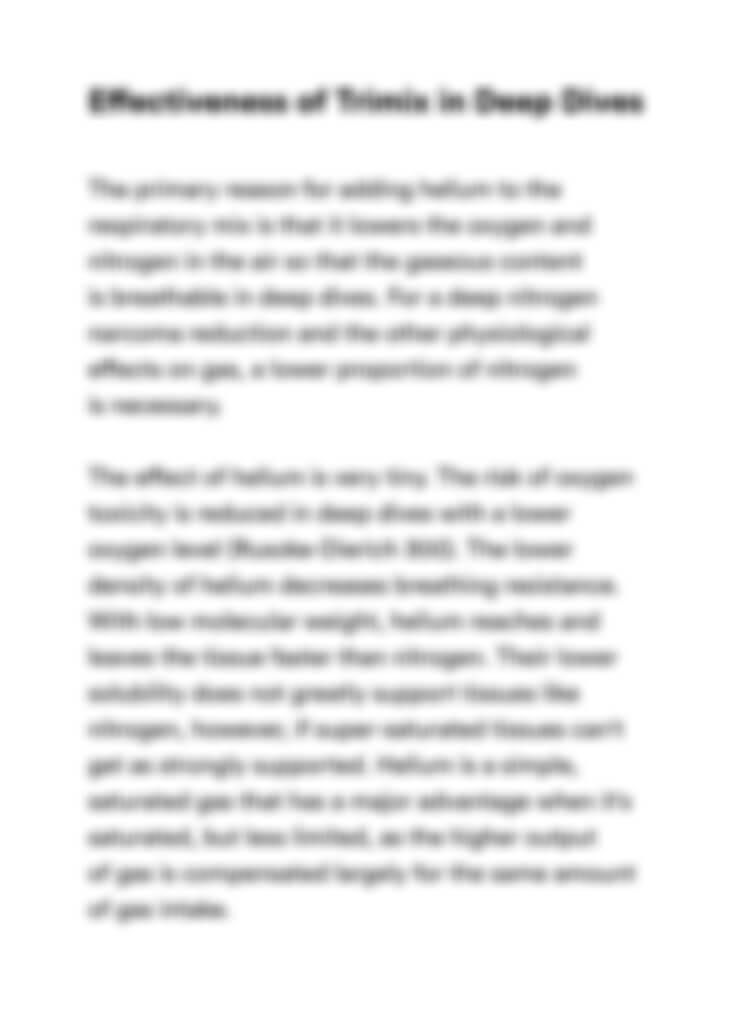Matlab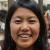Young H
5Project#1Young H
5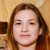LitTeacher1
4.5processor organization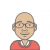masterwryta
0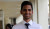pmwai337
0